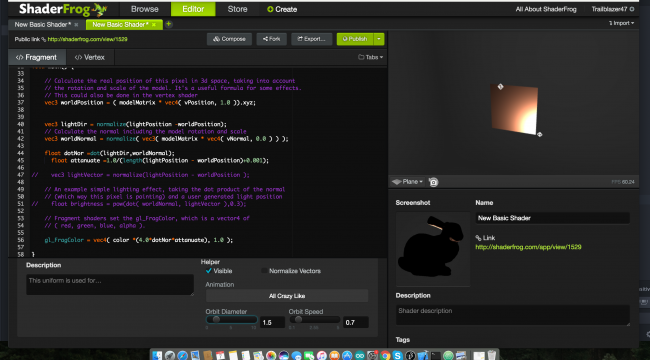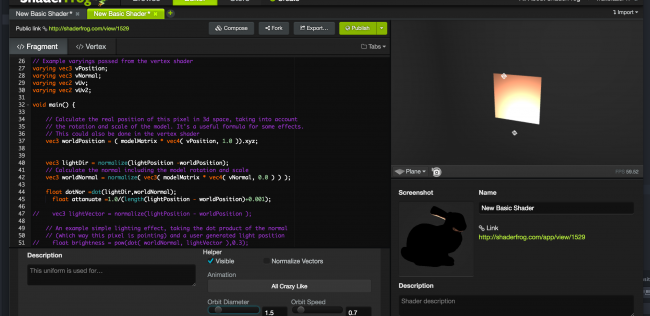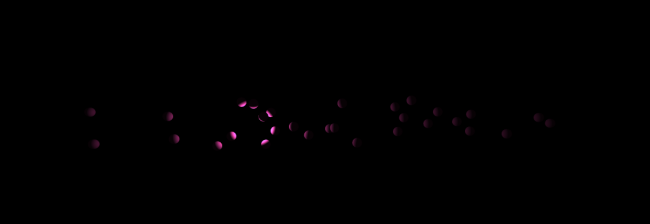#### Howdy, Stranger!

We are about to switch to a new forum software. Until then we have removed the registration on this forum.

# GLSL shader does not reproduce

edited June 2017

I am trying to make a new shader I have successfull(here it is ) made the visual I wanted in shaderFrog online shader tool. I also ported it to processing sketch but some thing is not write it does not create the same effect.

//code

``````PShader blast;
void setup()
{
size(700,700,P3D);

}
float t=0;
float r=110.0; //just 10pixels  away from sphere to test brightness effect
void draw()
{
t+=0.01;
background(0);
translate(width/2,height/2,0);
blast.set("lightPosition",r*sin(t*10) , 10.0,60.0);

noStroke();
fill(255,20,20);

sphere(100);
//box(600,600,1500);
}
``````

``````uniform mat4 transform;
uniform mat3 normalMatrix;
uniform mat4 texMatrix;
uniform mat4 modelview;

uniform vec3 lightPosition;

attribute vec4 position;
attribute vec4 color;
attribute vec3 normal;
attribute vec2 texCoord;

varying vec4 vertColor;
varying vec3 vNormal;
varying vec3 vPosition;
varying vec3 lightDir;
varying vec3 lpos;

void main() {
vec3 LightPos   = vec3(0., 500., 0.);
gl_Position = transform * position;
vec4 mecPosition =  modelview * vec4(position.xyz,1.0);
vPosition = mecPosition.xyz;
vNormal =  normal;
lightDir = normalize(lightPosition.xyz - vPosition);
vertColor = color;
lpos =lightPosition;
//vertTexCoord = texMatrix * vec4(texCoord, 1.0, 1.0);
}
``````

``````#ifdef GL_ES
precision mediump float;
precision mediump int;
#endif

varying vec4 vertColor;
varying vec3 ecNormal;
varying vec3 lightDir;

varying vec3 lpos;
varying vec3 vPosition;
uniform mat4 modelview;
uniform mat3 normalMatrix;
varying vec3 vNormal;

void main() {
vec3 direction = normalize(lightDir);
//vec3 normal = normalize(ecNormal);
//float intensity = max(0.0, dot(direction, normal));
//vec4 tintColor = vec4(intensity, intensity, intensity, 1) * vertColor;
vec3 Normal =normalMatrix * vNormal;
float dotNor =dot(direction,Normal);
float attanuate =1.0/ (length(lpos-vPosition )+0.001);
//  vec3 lightVec = normalize(lpos-vPosition);

//*(4.0*dotNor*attanuate);

gl_FragColor = vec4( vertColor.xyz *( 1.0 * dotNor* attanuate),1.0);
}
``````

I guess I have messed you with matrix I don't understand them right now exactly. Some Questions are lightPositions already transformed? I really wanted to do attenuation from light distance there is some thing wrong there because if remove it from main equation things appear but not that highlighted surface. also you can see the original shader I wrote in shader frog. I want that highlight when light is close to surface. that burning white.• edited June 2017

@trailbalzer47 try it with something simpel.

• edited June 2017

@trailbalzer47

I have also encountered problems with modelview.

this might also work:
`myshader.set("modelview",((PGraphicsOpenGL)g).modelview);`

• :( every try to create something nice fails ..I am learning shaders and all effects i can create with it but seems like processing is not able to handle things or some of the things are hidden. well did you figured our what could be the problem with porting that shader I wrote on shader frog to processing? its really simple shader .

• edited June 2017 Answer ✓

# start simpel

``````PShader blast;
void setup() {
size(400, 400, P3D);
noStroke();
blast.set("ucolor", 39/255f, 174/255f, 185/255f);
}
void draw() {
background(127,127,127);
float c=cos((frameCount*.01f))*400f, s=sin((frameCount*.01f))*400f, z=-200f;
blast.set("lightPos", c, s, z);
translate(200f, 200f, z);
sphere(100);
translate(c, s, z);
sphere(20);
}
````````````#ifdef GL_ES
precision mediump float;
precision mediump int;
#endif

varying vec4 vertColor;
varying vec3 ecNormal;
varying vec3 lightDir;
varying float dist;
void main() {
vec3 direction = normalize(lightDir);
vec3 normal = normalize(ecNormal);
float intensity = max(0.0, dot(direction, normal));
float att =1.0/(1.0+2.0*dist);
gl_FragColor = vec4(vertColor.xyz*(0.03 +120.30*intensity*att), 1.0) ;
}
``````

``````uniform mat4 modelview;
uniform mat4 transform;
uniform mat3 normalMatrix;

uniform vec4 lightPosition;
uniform vec3 lightNormal;

attribute vec4 position;
attribute vec4 color;
attribute vec3 normal;

varying vec4 vertColor;
varying vec3 ecNormal;
varying vec3 lightDir;
varying float dist;

void main() {
gl_Position = transform * position;
vec3 ecPosition = vec3(modelview * position);

ecNormal = normalize(normalMatrix * normal);
lightDir = normalize(lightPosition.xyz - ecPosition);
dist =length(lightPosition.xyz - ecPosition);
vertColor = color;
}
``````

code to test it (feels like moving light and attanuates at distance)

`

``````PShader blast;
void setup()
{
size(1300,700,P3D);
smooth(8);
displayDensity(1);
//sphereDetail(50);
initRand(); //intialize random positions for boxes
}
float t=0;
float r=0.0; //just 10pixels  away from sphere to test brightness effect
void draw()
{
r+=2;
// r=100.0+mouseX/1.0;
t+=0.01;
background(0);
translate(width/2,height/2,0);
//blast.set("lightPosition", r*sin(t),0.0 , r*cos(t));

noLights();

pointLight(255,255,255, r-width/2,0+30*(noise(t*10)-0.5) , 0  );
noStroke();
fill(#FF4BB7);

randomBoxes();  //create random boxes to visualise light movement

if(r>width)
r=-100;

println(frameRate);
}
int num=3000;
PVector[] pos=new PVector[num];
void initRand()
{
for(int i=0;i<num;i++)
{
pos[i] =new PVector(random(200,1000),random(50,650),random(-200,200));
}
}
void randomBoxes()
{
for(int i=0;i<num;i++){

pushMatrix();
translate(pos[i].x-width/2,pos[i].y-height/2,pos[i].z);
pushMatrix();
rotateX(2*PI*pos[i].x/2);
rotateY(2*PI*pos[i].y/2);
//sphere(8);
box(20,20,20);
popMatrix();
popMatrix();
}
}
``````

`

• edited June 2017

@trailbalzer47 great scetch, i will play with it, - later

just to make sure we on the same page:

from 0.0 to 1.0
`float att =1.0/(1.0+2.0*dist);`

from 0 to window.height
`translate(viewport.x,viewport.y,viewport.z);`• thanks but I tweeked your shader it still does not produce highlights don't know why?

` #version 150

``````in vec3 vPosition,vNormal;

uniform mat4 modelview;
uniform vec3 ucolor,lightPos;

out vec4 fragColor;

void main() {

vec3 worldPosition = ( modelview * vec4( vPosition, 1.0 )).xyz;
vec3 lightDir = normalize(lightPos -worldPosition);
vec3 worldNormal = normalize( vec3( modelview * vec4( vNormal, 0.0 ) ) );

float dotNor =max(0.0,dot(lightDir,worldNormal) );

//
float dist= length(lightPos - worldPosition);
float att =1.0/(1.0+2.0*dist);
fragColor = vec4(ucolor*1400.0*dotNor*att, 1.0 );
}
``````

` #version 150

``````in vec3 position,normal;

uniform mat4 projection,modelview;

out vec3 vPosition,vNormal;

void main() {
vNormal = normal;
vPosition = position;
gl_Position = projection*modelview* vec4( position, 1.0 );
}
``````

`

my shader that produce results shown in below image

```````
uniform mat4 modelview;
uniform mat4 transform;
uniform mat3 normalMatrix;

uniform vec4 lightPosition;
uniform vec3 lightNormal;

attribute vec4 position;
attribute vec4 color;
attribute vec3 normal;

varying vec4 vertColor;
varying vec3 vPosition;
varying vec3 ecNormal;
varying vec3 lightDir;
varying float dist;
varying vec3 ligpos;

void main() {
gl_Position = transform * position;

vPosition =vec3(modelview * position);
ecNormal = normalize(normalMatrix * normal);
lightDir = normalize(lightPosition.xyz - vPosition);
ligpos = lightPosition.xyz;
dist =length(lightPosition.xyz - vPosition);
vertColor = color;
}
`
``````

``````    `
#ifdef GL_ES
precision mediump float;
precision mediump int;
#endif

varying vec4 vertColor;
varying vec3 ecNormal;
varying vec3 vPosition;
varying float dist;
varying vec3 ligpos;
varying vec3 lightDir;
void main() {
vec3 direction = normalize(lightDir);
vec3 normal = normalize(ecNormal);
float intensity = max(0.0, dot(direction, normal));

float att =1.0/(1.0+2.0*dist);
float dist= length(ligpos-vPosition);
gl_FragColor = vec4(vertColor.xyz*(0.03 +120.30*intensity*att), 1.0) ;
}

`
``````

I am happy with this result .. I am going to make flocking simulation in 3d with this shader as light source around which flocking would occur hope so to show you final result. thanks again. seems like you are the only guy active on forum. ;-) great to see you again.• edited June 2017

@trailbalzer47 not sure why you use shaderfrog

https://processing.org/reference/directionalLight_.html

### Nice looking forward to see results. Keep on diggin it• @trailbalzer47

# thx for inspiration

https://www.openprocessing.org/sketch/433324• Great. I just use shaderfrog to make shaders and test the results .. it gives instant result while we are typing code. I loved it. besides I can easly port from it .. shaderToy is also good but for 2D and has perdefined notions of iRosolution etc..

Some how I love shaderfrog. thats it I just new about it a week ago. And I made some interesting(interesting to me atleast) shaders.

what inspired me to explore this lighting was

Its code is in Cinder and robert has it on his git. all shaders I tried recreating his shaders but don't get the way it looks in his video. also images create problems in 3D. he use glowing billboard for the lantern and lantern looks like burning. Some how his video is not great quality to see the details of lantern.

Thanks many how I do more of it. I will today make swarming bodies .. but I am trying to make my own shape with PShape for birds of boids . Or will use blender for it. see you soon.

• I maded flocking simulation in processing used multithreading but its so slow I can't put more than 500-600 particles .so I did not try the shader thing. but flocking is cool, I ported it from Cinder Tutorial code. here is the video.